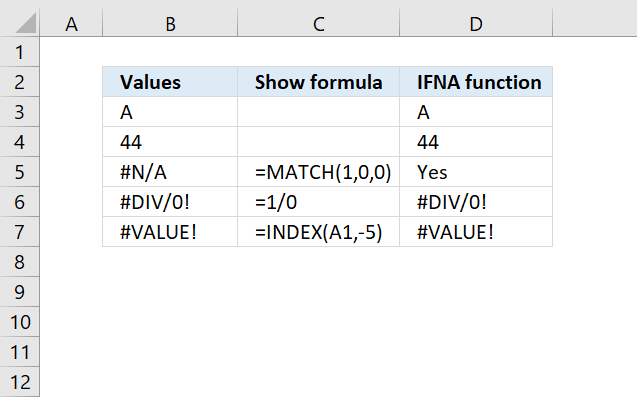Author: Oscar Cronquist Article last updated on May 04, 2022The IFNA function handles #N/A errors only, it returns a given value you specify if the formula returns a #N/A error.

## 1. IFNA Function Syntax

IFNA(value, value_if_na)

## 2. IFNA Function Arguments

 value Required. The value you want to check. value_if_na Required. The value to return if the value argument returns #N/A.

## 3. IFNA Function ExampleThe image above shows values and formulas in column B, the first cell B3 contains value A. The IFNA Function returns "A" because the result is not an #N/A error.

Formula in cell D3:

=IFNA(B3, "Yes")

#N/A means not available and is returned if a formula or function can't find a value. Cell B5 contains this formula:

=MATCH(1,0,0)

It returns a #N/A error because 1 is not found in the second argument in the MATCH function. The IFNA function catches this error and returns "Yes" which is the value we specified in the second argument.